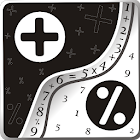Math Solver Mental calculation

All Android applications categories

All Android games categories# Math Solver Mental calculation

85 6.8

6.8 Users
rating

## Screenshots

Description

The application is based on mathematical arithmetic and working with numbers and colors. Constant exercise will help you improve and maintain your mental faculties. Classes with the program will help you develop and strengthen logical thinking, numerical fluency, memory and mindfulness. Enhances mathematical skills on addition, subtraction, multiplication, division.

The program features three types of exercises.

* The first is the usual mathematical arithmetic. In this type of exercise, you need to calculate the mathematical expression in the mind. The complexity of the expression can be adjusted. An example of the expression: (23 * 12) + (434 / 14) to evaluate the expression we get the answer: 307. The decision is given unlimited time.

* The second exercise on the development of memory. In this type of exercise, you need to calculate mathematical expressions sequentially displayed on the screen. Each successive term is calculated based on the previous one. Complexity of expression and time display on the screen can be adjusted. Example order: "10 + 2", "X / 2", "X - 3". First calculate the first expression: 10 + 2 = 12. Then, in the second expression is substituted in place of X 12 and we get the expression: 12 / 2 = 6. Remember the answer: 6. The third expression is substituted in place of X 6 and obtain the expression: 6 - 3 = 3. The final answer: 3.

* The third exercise on the development of mindfulness. In this type of exercise, you need to calculate mathematical expressions sequentially displayed on the screen. The expression is shown for a short time - you need to have time to recognize and remember. Among the featured numbers you need to see a mathematical operator (use the "+" and "-"), the operator determines the color of significant digits, the remaining digits just distracting noise. Then the numbers are hidden - and you need to evaluate the expression. Each successive term is calculated based on the previous one. The complexity of the expression, the time display on the screen and the time allotted for the solution can be adjusted. Example of a "3 + 5 1 2", " - 5 1 1 2", "2 7 5 4 + ". At first look the color of the addition operator ("+" - say the color red), then in the expression, select all of the red numbers (for example it will be 3 and 1) - remember the result. In the second expression say the subtraction yellow ("-"), select all the yellow numbers (for example it will be 1 and 2) - evaluate the expression: 31 - 12 = 19 - remember the resulting number (19). The following terms are also looking for the addition ("+") and by its color, select all the figures of the same color (for example, it will be 2 and 5), and then evaluate the expression: 19 + 25 = 44. The final answer: 44.

This information can also be obtained in the help of program.

Tags: calculator expression solver logic in , math solver , Устный счет * Усложненный скачать , Устный счет * Усложненный скачать , программа на javascript устный счет , тренировка устного счета , устный счет javascript , устный счет для андроид

from 85 reviews

"Good"

6.8